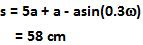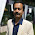## Sunday, June 6, 2010

### Irodov Problem 4.8We know that the velocity of a simple harmonic oscillator is given by,Hence, comparing with (1) we have,The period of the oscillations is given by,The position of the particle is given by,As seen in the figure, every 0.5 seconds the particle moves covers a distance of a, and hence in 2.5 seconds the particle will cover a distance of 5a. In the remaining 0.3 seconds, the particle will travel a distance of(as in Problem 4.7).

Hence the total distance traveled will be,1.1.2.